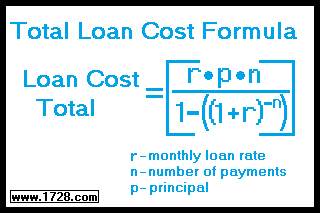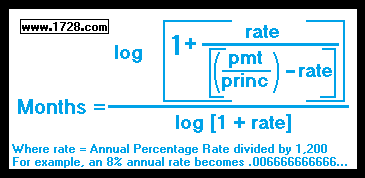Total Loan Cost Formula and Calculator
Scroll down for the Calculator

 Solving For Loan Cost TotalUse the above formula to determine the total amount you will pay for a loan. Example: If we borrow \$100,000 for 10 years at 8 per cent annual percentage rate, what is the total cost of the loan (principal plus interest) ? 1) The rate (r) would be 8 divided by 1,200       which equals .0066666666... 2) The number of payments (n) would be       12 months × 10 years = 120 payments. 3) So the total cost of the loan would be:
.0066666666   ×   \$100,000     ×   120
1 - (1 + .0066666666) -120

Which equals:

[ .0066666666   ×   \$100,000     × 120 ]   ÷   [ 1 - (1 + .0066666666) -120 ]     =

[ 80,000 ]   ÷   [ 1 - (0.45052346071062) ]     =

80,000   ÷   0.54947653928939   =

\$145,593.11

 Do you want to solve for: or or Input should only consist of numbers and decimal points. Do NOT use commas, dollar signs or per cent signs. >>>>> >>>>> >>>>>

* * * * * * * * * * * * * * * * * * * *

Solving For PrincipalThe above formula is used to calculate the principal of a loan if you know the total cost, interest rate and number of payments.

We'll use the previous data.
We have paid a total of \$145,593.11 for a 10 year loan at 8% interest.
What was the principal?
Using the above formula, the principal would be:

145,593.11 -145,593.11 × (1.00666666666666)-120
.00666666666666 × 120

Which Equals:

145,593.11 -(145,593.11 × 0.450523460710793)   =
.8

145,593.11 -65,593.11   =
.8

100,000.00

* * * * * * * * * * * * * * * * * * * *

Solving For Number of Payments (Months)The above formula is used to calculate how many months it will take to pay off a loan if you know the principal, interest rate and the monthly payment.

We'll use the previous data.
We are paying \$1,213.28 per month for a \$100,000.00 loan at 8% annual interest.
How many months will it take to pay for this loan?

Looking at the formula, we see the numerator equals:

log (1 + {.006666666666 / [(1,213.28 / \$100,000.00) -.006666666666]})
= log (1 + {.006666666666 / [0.0121328 -.006666666666]})
= log (1 + {.006666666666 / 0.005466133334})
= log (1 + 1.21963118338135)
= log (2.21963118338135)
numerator = 0.34628081755

denominator = log (1.0066666666)
denominator = .0028856882372

So, the time it will take equals the numerator divided by the denominator =
0.34628081755 / .0028856882372 =
120 months

RETURN   TO   HOME   PAGE